## Basics of Image Classification Techniques in Machine Learning

### What is Image Classification?

Classification between objects is a fairly easy task for us, but it has proved to be a complex one for machines and therefore image classification has been an important task within the field of computer vision.
Image classification refers to the labeling of images into one of a number of predefined classes.
There are potentially n number of classes in which a given image can be classified. Manually checking and classifying images could be a tedious task especially when they are massive in number (say 10,000) and therefore it will be very useful if we could automate this entire process using computer vision.

Some examples of image classification include:

• Labeling an x-ray as cancer or not (binary classification).
• Classifying a handwritten digit (multiclass classification).
• Assigning a name to a photograph of a face (multiclass classification).

The advancements in the field of autonomous driving also serve as a great example of the use of image classification in the real-world. For example, we can build an image classification model that recognizes various objects, such as other vehicles, pedestrians, traffic lights, and signposts on the road.

Now that we have a fair idea of what image classification comprises of, let’s start analyzing the image classification pipeline.

### Structure of an Image Classification Task

1. Image Preprocessing – The aim of this process is to improve the image data(features) by suppressing unwanted distortions and enhancement of some important image features so that our Computer Vision models can benefit from this improved data to work on.
2. Detection of an object – Detection refers to the localization of an object which means the segmentation of the image and identifying the position of the object of interest.
3. Feature extraction and Training– This is a crucial step wherein statistical or deep learning methods are used to identify the most interesting patterns of the image, features that might be unique to a particular class and that will, later on, help the model to differentiate between different classes. This process where the model learns the features from the dataset is called model training.
4. Classification of the object – This step categorizes detected objects into predefined classes by using a suitable classification technique that compares the image patterns with the target patterns.

Let’s discuss the most crucial step which is image preprocessing, in detail!

### Image Pre-processing

Pre-processing is a common name for operations with images at the lowest level of abstraction — both input and output are intensity images.

Need for Image-Preprocessing
Computers are able to perform computations on numbers and is unable to interpret images in the way that we do. We have to somehow convert the images to numbers for the computer to understand.
The aim of pre-processing is an improvement of the image data that suppresses unwilling distortions or enhances some image features important for further processing.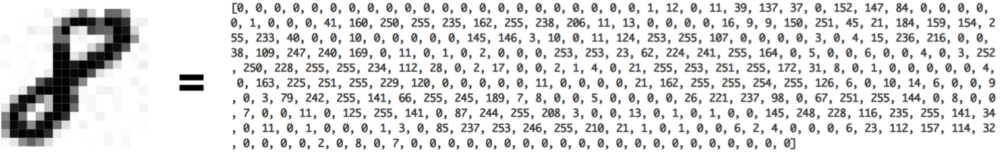How computers see an ‘8’

Steps for image pre-processing:

• Resize image
• Data Augmentation
• Gray scaling of image
• Reflection
• Gaussian Blurring
• Histogram Equalization
• Rotation
• Translation

Step 1
In this step, we simply store the path to our image dataset into a variable and then we create a function to load folders containing images into arrays so that computers can deal with it.

Sample code for reading an image dataset with 2 classes:

``````# importing libraries
from pathlib import Path
import glob
import pandas as pd

images_dir = Path('img')
images = images_dir.glob("*.tif")

train_data = []

counter = 0
for img in images:
counter += 1
if counter <= 130:
train_data.append((img,1))
else:
train_data.append((img,0))

# converting data into pandas dataframe for easy visualization
train_data = pd.DataFrame(train_data,columns=['image','label'],index = None)
```PythonCopy```

Step 2.
Resize image
Some images captured by a camera and fed to our AI algorithm vary in size, therefore, we should establish a base size for all images fed into our AI algorithms by resizing them.

Sample code for resizing images into 229×229 dimensions:

``````img = cv2.resize(img, (229,229))
```PythonCopy```

Step 3
Data Augmentation
Data augmentation is a way of creating new ‘data’ with different orientations. The benefits of this are two-fold, the first being the ability to generate ‘more data’ from limited data and secondly, it prevents overfitting.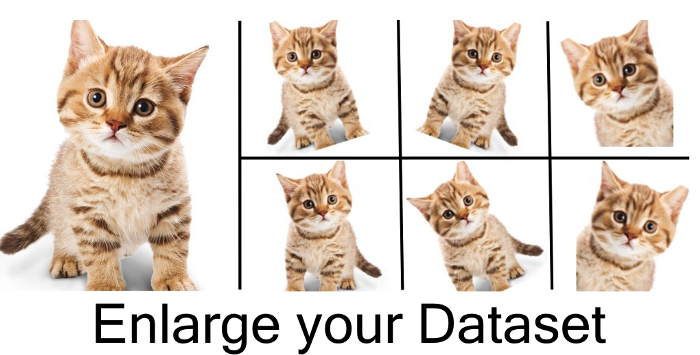Data Augmentation Techniques:

1. Gray Scaling
The image will be converted to gray scale (range of gray shades from white to black) the computer will assign each pixel a value based on how dark it is. All the numbers are put into an array and the computer does computations on that array.

Sample code to convert an RGB(3 channels) image into a Gray scale image:

``````import cv2
img = cv2.cvtColor(img, cv2.COLOR_BGR2GRAY)
```PythonCopy```

RGB ImageGrayscale Image: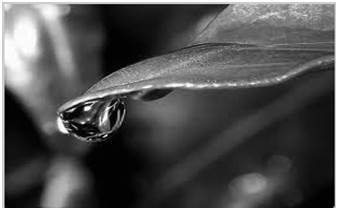1. Reflection/Flip
You can flip images horizontally and vertically. Some frameworks do not provide function for vertical flips. But, a vertical flip is equivalent to rotating an image by 180 degrees and then performing a horizontal flip.

Sample Code:

``````# horizontal flip
img = cv2.flip(img, 0)

# vertical flip
img = cv2.flip(img,1)
```PythonCopy```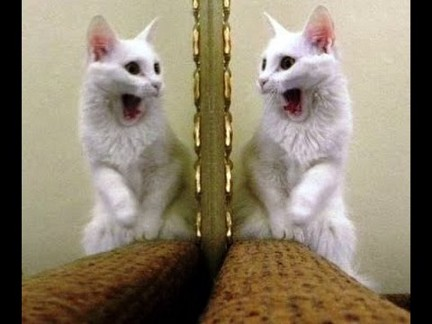Image showing horizontal reflection

1. Gaussian Blurring
Gaussian blur (also known as Gaussian smoothing) is the result of blurring an image by a Gaussian function. It is a widely used effect in graphics software, typically to reduce image noise.

Sample Code:

``````from scipy import ndimage
img = ndimage.gaussian_filter(img, sigma= 5.11)
```PythonCopy```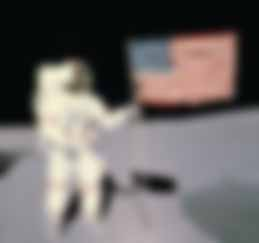Image with blur radius = 5.1

1. Histogram Equalization
Histogram equalization is another image processing technique to increase global contrast of an image using the image intensity histogram. This method needs no parameter, but it sometimes results in an unnatural looking image.

Sample Code

``````# histogram equalization function
def hist(img):
img_to_yuv = cv2.cvtColor(img,cv2.COLOR_BGR2YUV)
img_to_yuv[:,:,0] = cv2.equalizeHist(img_to_yuv[:,:,0])
hist_equalization_result = cv2.cvtColor(img_to_yuv, cv2.COLOR_YUV2BGR)
return hist_equalization_result
```PythonCopy```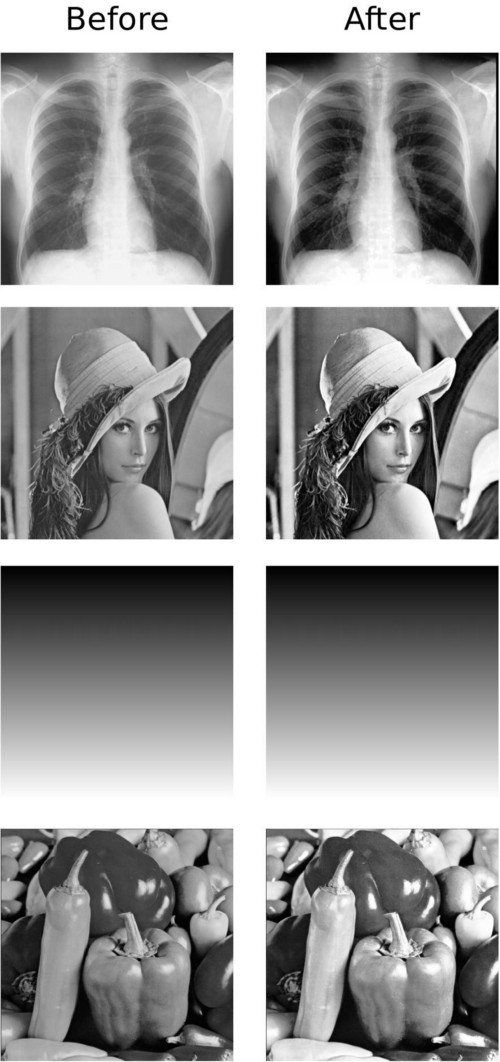1. Rotation
This is yet another image augmentation technique. Rotating an image might not preserve its original dimensions (depending on what angle you choose to rotate it with )

Sample Code

``````import random
# function for rotation
def rotation(img):
rows,cols = img.shape,img.shape
randDeg = random.randint(-180, 180)
matrix = cv2.getRotationMatrix2D((cols/2, rows/2), randDeg, 0.70)
rotated = cv2.warpAffine(img, matrix, (rows, cols), borderMode=cv2.BORDER_CONSTANT,borderValue=(144, 159, 162))
return rotated
```PythonCopy```The images are rotated by 90 degrees clockwise with respect to the previous one, as we move from left to right.

1. Translation
Translation just involves moving the image along the X or Y direction (or both).
This method of augmentation is very useful as most objects can be located at almost anywhere in the image. This forces our feature extractor to look everywhere.

Sample Code

``````img = cv2.warpAffine(img, np.float32([[1, 0, 84], [0, 1, 56]]), (img.shape, img.shape),
borderMode=cv2.BORDER_CONSTANT,borderValue=(144, 159, 162))
```PythonCopy```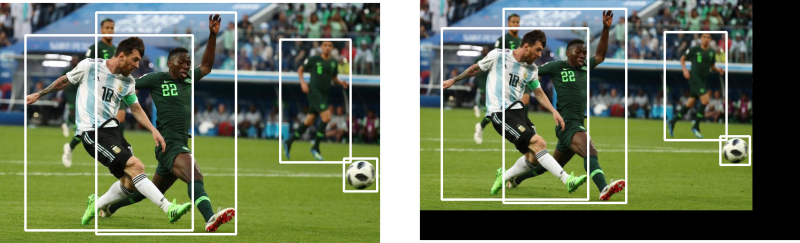## Image Classification Techniques

We will start with some statistical machine learning classifiers like Support Vector Machine and Decision Tree and then move on to deep learning architectures like Convolutional Neural Networks.

To support their performance analysis, the results from an Image classification task used to differentiate lymphoblastic leukemia cells from non-lymphoblastic ones have been provided. The features have been extracted using a convolutional neural network, which will also be discussed as one of our classifiers. This is because deep learning models have achieved state of the art results in the feature extraction process.

Different classifiers are then added on top of this feature extractor to classify images.

## 1. Support Vector Machines

It is a supervised machine learning algorithm used for both regression and classification problems.
When used for classification purposes, it separates the classes using a linear boundary.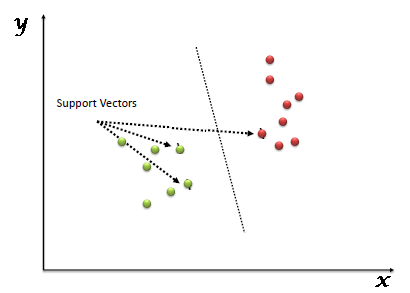It builds a hyper-plane or a set of hyper-planes in a high dimensional space and good separation between the two classes is achieved by the hyperplane that has the largest distance to the nearest training data point of any class.
The real power of this algorithm depends on the kernel function being used.
The most commonly used kernels are:

• Linear Kernel
• Gaussian Kernel
• Polynomial Kernel

Code Snippet:

This is the base model/feature extractor using Convolutional Neural Network, using Keras with Tensorflow backend

``````model = Sequential()

model_feat = Model(inputs=model.input,outputs=model.get_layer('dense_1').output)
feat_train = model_feat.predict(X_train)
```PythonCopy```

Fitting of SVM as a classifier

``````svm = SVC(kernel='rbf')
svm.fit(feat_train,np.argmax(y_train,axis=1))

svm.score(feat_test,np.argmax(y_test,axis=1))
```PythonCopy```

Accuracy score on test data: 85.68

## 2. Decision Trees

It is also a supervised machine learning algorithm, which at its core is the tree data structure only, using a couple of if/else statements on the features selected.
Decision trees are based on a hierarchical rule-based method and permits the acceptance and rejection of class labels at each intermediary stage/level.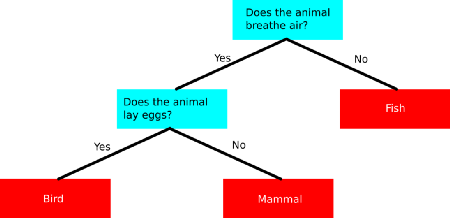This method consists of 3 parts:

• Partitioning the nodes
• Finding the terminal nodes
• Allocation of the class label to terminal node

Code
Feature Extractor

``````model = Sequential()

model_feat = Model(inputs=model.input,outputs=model.get_layer('dense_2').output)
feat_train = model_feat.predict(X_train)
```PythonCopy```

Decision Tree Classifier

``````dt = DecisionTreeClassifier(criterion = "entropy", random_state = 100,max_depth=3, min_samples_leaf=5)
dt.fit(feat_train,np.argmax(y_train,axis=1))

dt.score(feat_test,np.argmax(y_test,axis=1))
```PythonCopy```

Accuracy on test set: 84.61

## 3. K Nearest Neighbor

The k-nearest neighbor is by far the most simple machine learning algorithm.
This algorithm simply relies on the distance between feature vectors and classifies unknown data points by finding the most common class among the k-closest examples.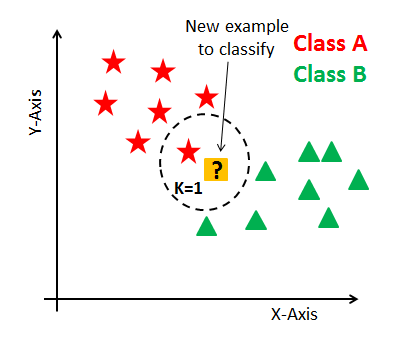Here we can see there are two categories of images and that each of the data points within each respective category are grouped relatively close together in an n-dimensional space.

In order to apply the k-nearest Neighbor classification, we need to define a distance metric or similarity function. Common choices include the Euclidean distance and Manhattan distance

Code
Base Model/feature extractor

``````model = Sequential()

model_feat = Model(inputs=model.input,outputs=model.get_layer('dense_2').output)
feat_train = model_feat.predict(X_train)
```PythonCopy```

KNN classifier

``````knn = KNeighborsClassifier(n_neighbors=12)
knn.fit(feat_train,np.argmax(y_train,axis=-1))

knn.score(feat_test,np.argmax(y_test,axis=1))
```PythonCopy```

Accuracy on test set: 86.32

## 4. Artificial Neural Networks

Inspired by the properties of biological neural networks, Artificial Neural Networks are statistical learning algorithms and are used for a variety of tasks, from relatively simple classification tasks to computer vision and speech recognition.
ANNs are implemented as a system of interconnected processing elements, called nodes, which are functionally analogous to biological neurons.The connections between different nodes have numerical values, called weights, and by altering these values in a systematic way, the network is eventually able to approximate the desired function.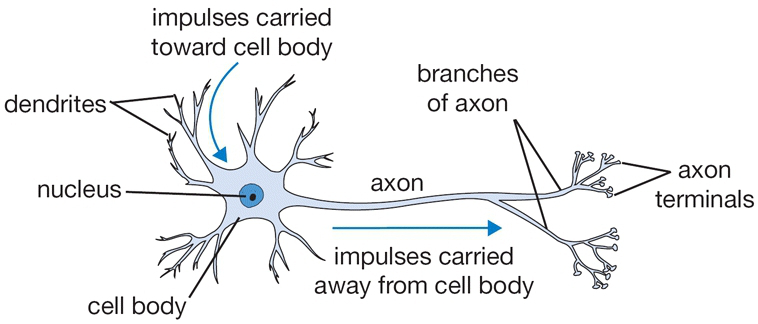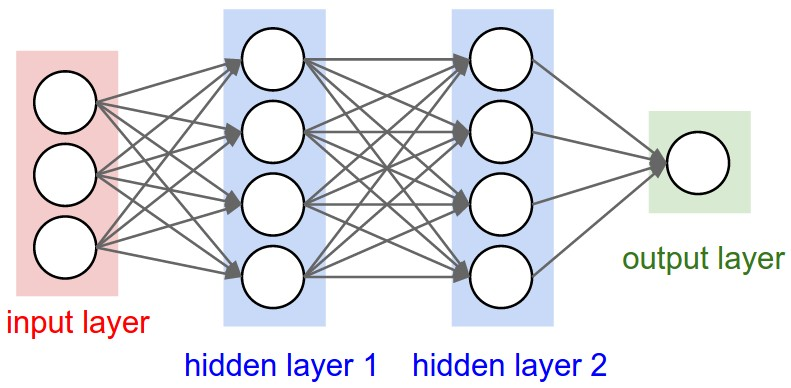The hidden layers can be thought of as individual feature detectors, recognizing more and more complex patterns in the data as it is propagated throughout the network. For example, if the network is given a task to recognize a face, the first hidden layer might act as a line detector, the second hidden takes these lines as input and puts them together to form a nose, the third hidden layer takes the nose and matches it with an eye and so on, until finally the whole face is constructed. This hierarchy enables the network to eventually recognize very complex objects.

Code
ANN as feature extractor using softmax classifier

``````model_ann = Sequential()

history = model_ann.fit(X_train, y_train,epochs=100,batch_size=100)
history
```PythonCopy```

Accuracy on test data: 83.1
This result has been recorded for 100 epochs, and the accuracy improves as the epochs are further increased.

Link to study ANN in detail

## 5. Convolutional Neural Networks

Convolutional neural networks (CNN) is a special architecture of artificial neural networks. CNNs uses some of its features of visual cortex and have therefore achieved state of the art results in computer vision tasks.

Let’s cover the use of CNN in more detail.

Convolutional neural networks are comprised of two very simple elements, namely convolutional layers and pooling layers.
Although simple, there are near-infinite ways to arrange these layers for a given computer vision problem.
The elements of a convolutional neural network, such as convolutional and pooling layers, are relatively straightforward to understand.
The challenging part of using convolutional neural networks in practice is how to design model architectures that best use these simple elements.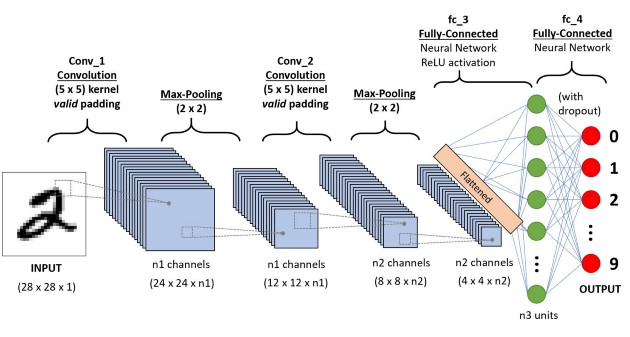Code
CNN as feature extractor using softmax classifier

``````model = Sequential()

batch_size = 100
epochs= 100

optimizer = keras.optimizers.rmsprop(lr = 0.0001, decay = 1e-6)

model.compile(loss = 'binary_crossentropy',optimizer = optimizer, metrics = ['accuracy',keras_metrics.precision(), keras_metrics.recall()])

history = model.fit(X_train,y_train,steps_per_epoch = int(len(X_train)/batch_size),epochs=epochs)
history
```PythonCopy```

Accuracy on test data with 100 epochs: 87.11
Since this model gave the best result amongst all, it was trained longer and it achieved 91% accuracy with 300 epochs.